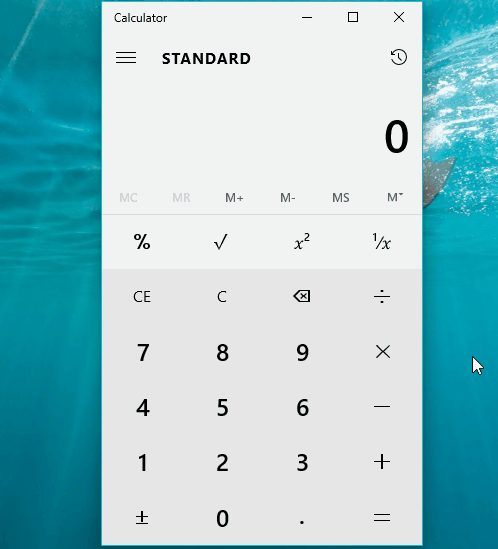# Calculator App In Windows 10 Doesn’t Know Maths, Does Horrible Calculations

Short Bytes: A Redditor has reported that there are some calculation issues with the new Calculator app present in Microsoft Windows 10 operating system. In Standard mode, the Calculator app displays wrong answers for basic calculations involving multiple operators.

The Windows 10 OS features a revamped calculator app. Microsoft made efforts to make it better than the previous and touted its instant calculation abilities. But the Windows calculator app defies the basic purpose of having a calculator at the first place because the app didn’t pay attention while attending math lectures at Microsoft’s campus.

A Redditor /u/EvilDoesIt has pointed out a horrible blunder in Windows Calculator. The app starts calculation as soon as you start entering the numbers and operator. For instance, if you do 3+3*3, the answer is 12. But in the calculator, when you start typing 3+3, it will add them. And when you proceed further with *3, it will multiply the outcome of 3+3 i.e. 6. Thus, 3+3*3 = 18 and not 12.

When you do 3*3+3, the answer would be 12 because the Calculator does the multiply operation first. The problem, however, occurs only in the Standard mode. If you switch to Scientific Mode, the Calculator app displays the correct answer.

I was curious enough to try it and got the unexpected answer.It appears as if the Windows Calculator app is confused about the order of operators in Standard Mode. It might be possible that the Redmond designed it that way. Hopefully, Microsoft would be quick to issue a fix.

I think, now we know why Microsoft named its latest OS Windows 10. Maybe, Microsoft bosses used the same calculator and got 8.1+1=10. 😅

If you have something to add, tell us in the comments below.